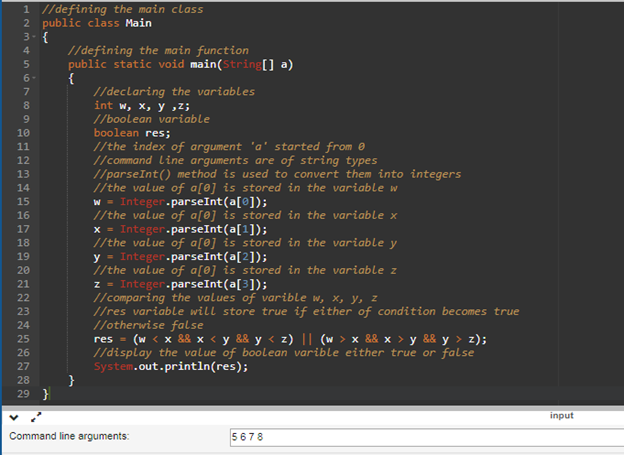# In java write a program that takes four int command-line arguments w, x, y, z,. define a boolean variable whose value is true if the four values are either in strictly acending order (w<x<y<z) or strictly descending order (w>x>y>z), and false otherwise, then display the boolean variable value.Note: Do not use if statements on the programNote: Assume that the inputs will always be integers

Question
2 views

In java write a program that takes four int command-line arguments w, x, y, z,. define a boolean variable whose value is true if the four values are either in strictly acending order (w<x<y<z) or strictly descending order (w>x>y>z), and false otherwise, then display the boolean variable value.

Note: Do not use if statements on the program

Note: Assume that the inputs will always be integers

check_circle

Step 1

The java program and its approach are given below: -

Approach: -

• Defining the main class and inside the main class defining the main() method.
• Inside the main() method, declaring the variable as per the given instructions.
• The variables store the value given as the command line arguments accordingly (description is given as the comments in the program).
• The Boolean variable will store true if any of the conditions is valid that is either the command line arguments are given in the ascending or descending order otherwise false.
• Displays the value stored in the Boolean variable.
Step 2

Java program: -

//defining the main class

public class Main

{

//defining the main method

public static void main(String[] a)

{

//declaring the variables

int w, x, y, z;

//boolean variable

boolean res;

//the index of argument 'a' started from 0

//command line arguments are of string types

//parseInt() method is used to convert them into integers

//the value at first index is stored in the variable w

w = Integer.parseInt(a);

//the value at second index is stored in the variable x

x = Integer.parseInt(a);

//the value at third index is stored in the variable y

y = Integer.parseInt(a);

//the value at fourth index is stored in the variable z

z = Integer.parseInt(a);

//comparing the values of varible w, x, y, z

//res variable will store true if either of condition becomes true

//otherwise false

res = (w < x && x < y && y < z) || (w > x && x > y && y > z);

//display the value of boolean varible either true or false

System.out.println(res);

}

}

Step 3

Program Screenshot with command-line arguments: -...

### Want to see the full answer?

See Solution

#### Want to see this answer and more?

Solutions are written by subject experts who are available 24/7. Questions are typically answered within 1 hour.*

See Solution
*Response times may vary by subject and question.
Tagged in

### Computer Science